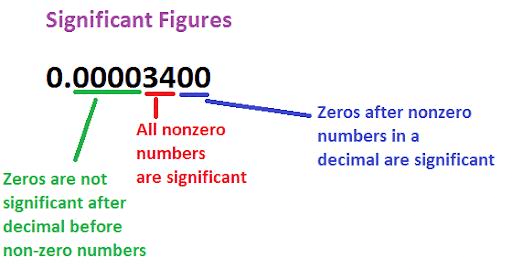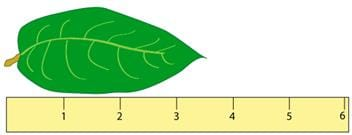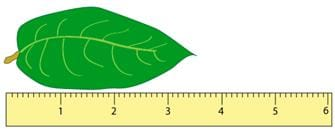NEET  >  Significant Figures

# Significant Figures Notes | Study Physics Class 11 - NEET

## Document Description: Significant Figures for NEET 2022 is part of Physics Class 11 preparation. The notes and questions for Significant Figures have been prepared according to the NEET exam syllabus. Information about Significant Figures covers topics like What are Significant Figures?, Order of Magnitude and Significant Figures Example, for NEET 2022 Exam. Find important definitions, questions, notes, meanings, examples, exercises and tests below for Significant Figures.

Introduction of Significant Figures in English is available as part of our Physics Class 11 for NEET & Significant Figures in Hindi for Physics Class 11 course. Download more important topics related with notes, lectures and mock test series for NEET Exam by signing up for free. NEET: Significant Figures Notes | Study Physics Class 11 - NEET
 Table of contents What are Significant Figures? Order of Magnitude
 1 Crore+ students have signed up on EduRev. Have you?

What are Significant Figures?It is important to understand that the way a measurement is taken affects its accuracy. For example, you could measure the length of a leaf with a ruler that had markings every centimeter (cm). In this example, illustrated below, the leaf is longer than 3 cm and shorter than 4 cm, so you might estimate that the leaf is 3.5 cm long.On the other hand, if you measured the same leaf with a ruler that had markings every millimeter (mm), as drawn below, you can see that the end of the leaf actually falls between the markings for 3.5 and 3.6 cm (or 35 and 36 mm). Because it’s closer to the 3.5 marking, you might estimate that the leaf is 3.52 cm (or 35.2 mm) long.Using the second ruler, it’s possible to estimate that the leaf is 3.52 cm long, but it is not possible to measure that accurately with the first ruler. In this way, the number of digits in the measured value gives us an idea of the maximum accuracy of the measurement. These are called significant digits or significant figures.

RULES FOR SIGNIFICANT FIGURES

• All non-zero numbers ARE significant. The number 33.2 has THREE significant figures because all of the digits present are non-zero.
• Zeros between two non-zero digits ARE significant. 2051 has FOUR significant figures. The zero is between a 2 and a 5.
• Leading zeros are NOT significant. They're nothing more than "place holders." The number 0.54 has only TWO significant figures. 0.0032 also has TWO significant figures. All of the zeros are leading.
• Trailing zeros to the right of the decimal ARE significant. There are FOUR significant figures in 92.00.
92.00 is different from 92: a scientist who measures 92.00 milliliters knows his value to the nearest 1/100th milliliter; meanwhile his colleague who measured 92 milliliters only knows his value to the nearest 1 milliliter. It's important to understand that "zero" does not mean "nothing." Zero denotes actual information, just like any other number. You cannot tag on zeros that aren't certain to belong there.
• Trailing zeros in a whole number with the decimal shown ARE significant. Placing a decimal at the end of a number is usually not done. By convention, however, this decimal indicates a significant zero.
For example, "540." indicates that the trailing zero IS significant; there are THREE significant figures in this value.
• Trailing zeros in a whole number with no decimal shown are NOT significant. Writing just "540" indicates that the zero is NOT significant, and there are only TWO significant figures in this value.
• Exact numbers have an INFINITE number of significant figures. This rule applies to numbers that are definitions.
For example, 1 meter = 1.00 meters
= 1.0000 meters
= 1.0000000000000000000 meters, etc.
So now back to the example posed in the Rounding Tutorial: Round 1000.3 to four significant figures. 1000.3 has five significant figures (the zeros are between non-zero digits 1 and 3, so by rule 2 above, they are significant.) We need to drop the final 3, and since 3 < 5, we leave the last zero alone. so 1000. is our four-significant-figure answer. (from rules 5 and 6, we see that in order for the trailing zeros to "count" as significant, they must be followed by a decimal. Writing just "1000" would give us only one significant figure.)
• For a number in scientific notation: N × 10x, all digits comprising N ARE significant by the first 6 rules; "10" and "x" are NOT significant. 5.02 × 104 has THREE significant figures: "5.02." "10 and "4" are not significant.
Rule 8 provides the opportunity to change the number of significant figures in a value by manipulating its form.
For example, let's try writing 1100 with THREE significant figures. By rule 6, 1100 has TWO significant figures; its two trailing zeros are not significant. If we add a decimal to the end, we have 1100., with FOUR significant figures (by rule 5.) But by writing it in scientific notation: 1.10 × 103, we create a THREE-significant-figure value.

Order of Magnitude

• Order of magnitude of a quantity is the power of 10 required to represent that quantity. This power is determined after rounding off the value of the quantity properly. For rounding off, the last digit is simply ignored if it is less than 5 and, is increased by one if it is 5 or more than 5.
• When a number is divided by 10(where x is the order of the number) the result will always lie between 0.5 and 5 i.e. 0.5 < N/10< 5

Example. Order of magnitude of the following values can be determined as follows:

(a) 49 = 4.9 × 101 » 101 \ Order of magnitude = 1

(b) 51 = 5.1 × 101 » 102 \ Order of magnitude = 2

(c) 0.049 = 4.9 × 10–2 » 10–2 \ Order of magnitude = –2

(d) 0.050 = 5.0 × 10–2 » 10–1 \ Order of magnitude = –1

(e) 0.051 = 5.1 × 10–2 » 10–1 \ Order of magnitude = –1

ROUNDING

When doing calculations using significant figures, you will find it necessary to round your answer to the nearest significant digit. There are therefore a few rules of rounding that help retain as much accuracy as possible in the final answer.

• Complete all sequential calculations BEFORE doing any rounding, since rounding early reduces the number of significant figures available for subsequent calculations.
• If the last significant digit is followed by a 6, 7, 8, or 9, round up.
Example: 5.677, rounded to three significant digits, is 5.68
• If the last significant digit is followed by a 0, 1, 2, 3, or 4, simply drop the trailing digits.
Example: 561200, rounded to three significant digits, is 561000
• If the last significant digit is EVEN, and is followed by a 5, drop the trailing digits.
Example: 45850, rounded to three significant digits, is 45800
• If the last significant digit is ODD, and is followed by a 5, round up.
Example: 3.47588, rounded to three significant digits, is 3.48

AMBIGUOUS ZEROS
So what happens if your calculation or measurement ends in a zero? For example, what if you measured a branch that was 200 cm (not 199 or 201 cm) long? The zeros in a measured value of 200 cm in this case appear ambiguous, since it could suggest that there is only one significant digit.
One way to reduce this ambiguity is to use significant figures with scientific notation.

The document Significant Figures Notes | Study Physics Class 11 - NEET is a part of the NEET Course Physics Class 11.
All you need of NEET at this link: NEET

## Physics Class 11

126 videos|449 docs|210 tests
 Use Code STAYHOME200 and get INR 200 additional OFF

## Physics Class 11

126 videos|449 docs|210 tests

### How to Prepare for NEET

Read our guide to prepare for NEET which is created by Toppers & the best Teachers

Track your progress, build streaks, highlight & save important lessons and more!

,

,

,

,

,

,

,

,

,

,

,

,

,

,

,

,

,

,

,

,

,

;